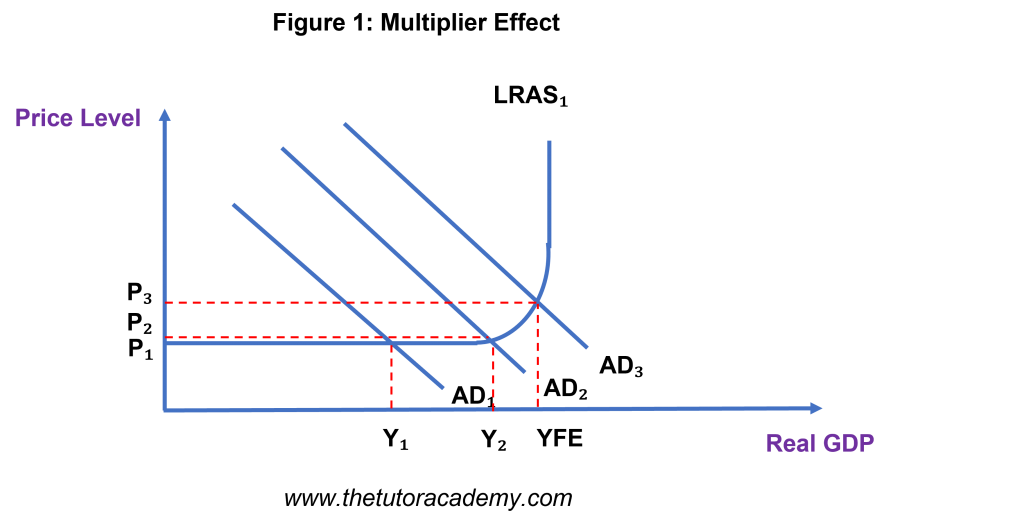# The Multiplier

Courses Info

Level: AS Levels, A Level, GCSE – Exam Boards: Edexcel, AQA, OCR, WJEC, IB, Eduqas – Economics Revision Notes

### The Multiplier Effect• The Multiplier Effect suggests that an increase in Aggregate Demand caused by an increase in Injections into the economy will result in greater National Income
• When an initial injection into the economy causes a larger increase in national income
• As the injections increase, the rise in national income will continue to get bigger
• If there are more withdrawals circulating in the economy, the multiplier effect will be smaller
• Marginal Propensity to Consume = change in consumption / change in income
• The more money you save and the less you spend, the lower the multiplier ratio will be
• The multiplier ratio decreases as the marginal propensity to save increases
• Marginal Propensity to Save (mps) – proportion of income a consumer saves rather than spends
• Marginal Propensity to Withdraw (mpw) – the extra income withdrawn from the circular flow and consists of tax, savings, imports
• Marginal Propensity to Tax (mpt) = change in tax / change in income
• Marginal Propensity to Import (mpm) = the amount imports increase or decrease as a result of a one unit change in income

### The Multiplier Ratio

• Multiplier (k) = Change in Real GDP / Change in Injections OR
• Multiplier (k) = 1 / (1 – MPC) = 1 / (1 – MPW)
• mpw = mpt + mpm + mps
• [numerical example]

### Negative Multiplier Effect

• If there is a cut in government spending, some public sector worker may experience a job loss
• Workers who have lost their job would have less disposable income to spend on purchasing goods / services
• Consequently, there will be less injections into the economy, causing an initial fall in national income
• This causes a negative multiplier effect to occur

Quick Fire Quiz – Knowledge Check

1. Define ‘Marginal Propensity to Consume’ (2 marks)

2. Define ‘Marginal Propensity to Withdraw’ (2 marks)

3. Define ‘Marginal Propensity to Save’ (2 marks)

4. Define ‘Marginal Propensity to Tax’ (2 marks)

5. Define ‘Marginal Propensity to Import’ (2 marks)

6. Explain what the Multiplier Effect shows (4 marks)

7. Identify the formulas to calculate the Multiplier Ratio (4 marks)

9. Explain what a Negative Multiplier Effect is (4 marks)

Next Revision Topics

A Level Economics Past PapersEconomics Tutor